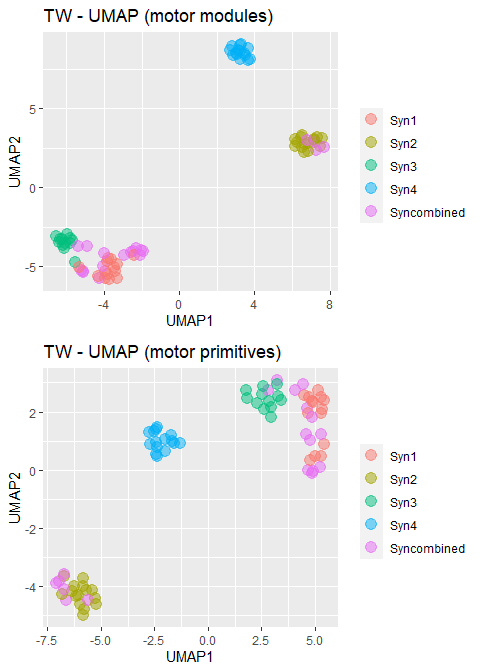# Plotting options in musclesyneRgies

library(musclesyneRgies)

## Plots help the workflow

To better control what is going on with the computation functions, plots are tremendously helpful. The first important plot is that of raw EMG.

# Load the built-in example data set
data("RAW_DATA")

# Raw EMG can be plotted with the following (the first three seconds are plot by default)
pp <- plot_rawEMG(
RAW_DATA[],
trial = names(RAW_DATA),
row_number = 4,
col_number = 4,
line_col = "tomato3"
)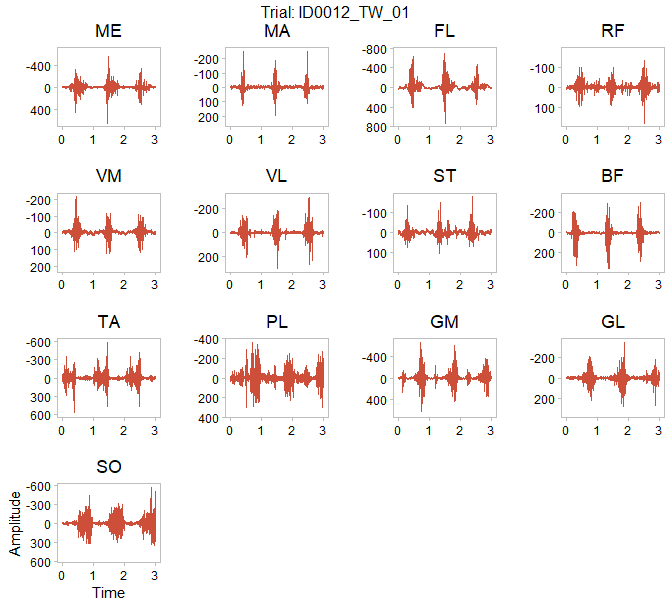The second important plot, is that of filtered and normalised EMG. The figure can be used to check the average activations across all available cycles and search for potential inconsistencies in the acquisition.

# Filter...
filtered_EMG <- lapply(RAW_DATA, function(x) filtEMG(x))
# ...and normalise raw EMG
norm_EMG <- lapply(
filtered_EMG,
function(x) {
normEMG(x,
trim = TRUE,
cy_max = 3,
cycle_div = c(100, 100)
)
}
)

# The filtered and time-normalised EMG can be plotted with the following
pp <- plot_meanEMG(
norm_EMG[],
trial = names(norm_EMG),
row_number = 4,
col_number = 4,
line_size = 0.8,
line_col = "tomato3"
)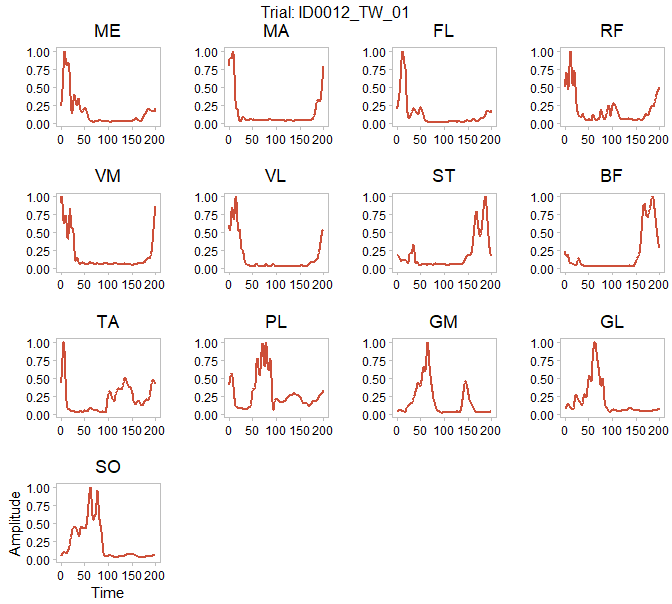Third, muscle synergies extracted from each trial can be plotted to eyeball the first (unclassified) results.

# Extract synergies via NMF
SYNS <- lapply(norm_EMG, synsNMF)

# The extracted synergies can be plotted with the following
pp <- plot_syn_trials(SYNS[],
max_syns = max(unlist(lapply(SYNS, function(x) x\$syns))),
trial = names(SYNS),
line_size = 0.8,
line_col = "tomato1",
sd_col = "tomato4"
)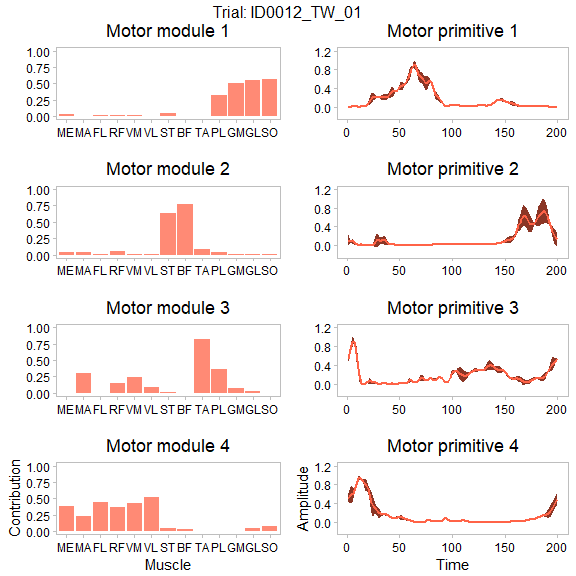Last but not least, classified synergies from all trials can be put into a single, final plot which resembles what we can usually find in motor control scientific publications.

# Load synergies
data("SYNS")

# Classify with k-means
# A plot of FWHM vs. CoA of the classified synergies appears by default
# This should help the user to identify potential malfunctions in the clustering
SYNS_classified <- classify_kmeans(SYNS)
# Classified synergies can be finally plotted with
pp <- plot_classified_syns(SYNS_classified,
line_col = "tomato1",
sd_col = "tomato4",
condition = "TW"
) # "TW" = Treadmill Walking, change with your own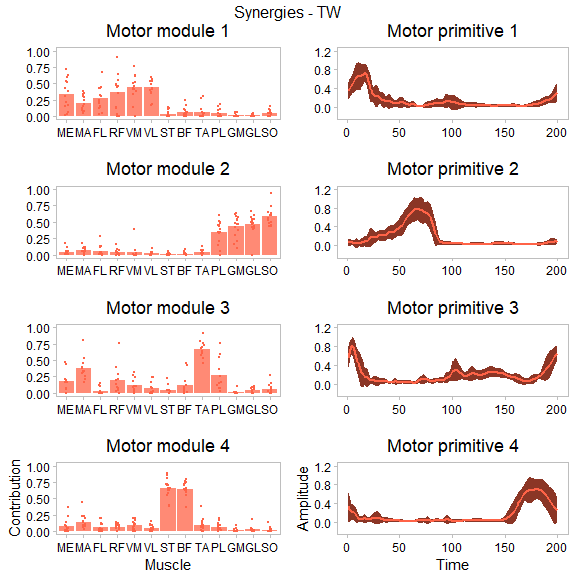An additional, almost purely cosmetic, tool is the 2D UMAP of the classified synergies. This can be of some help in visually identifying the classified clusters of similar synergies, but it should be used only for data inspection purposes and not to drive the whole analysis.

pp <- plot_classified_syns_UMAP(
SYNS_classified,
condition = "TW"
)